# | 10. The electrolyte in automobile lead batteries is a 3.75M sulfuric acid (H,SO,)solution. Calculate the following if density of the solution is 1.234 g/mLa) Mass percents of solute and solvent.b) Molalityc) Mole fractionssolute and solvent.

Question
18 views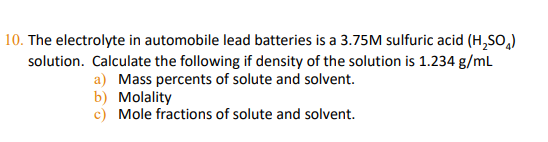help_outlineImage Transcriptionclose| 10. The electrolyte in automobile lead batteries is a 3.75M sulfuric acid (H,SO,) solution. Calculate the following if density of the solution is 1.234 g/mL a) Mass percents of solute and solvent. b) Molality c) Mole fractions solute and solvent. fullscreen
check_circle

Step 1

(a)Calculate mass percent of solute and solvent:

The molarity of sulfuric acid is 3.75 M. It implies that 1 L of solution contains 3.75 moles of sulfuric acid.

1 L = 1000 mL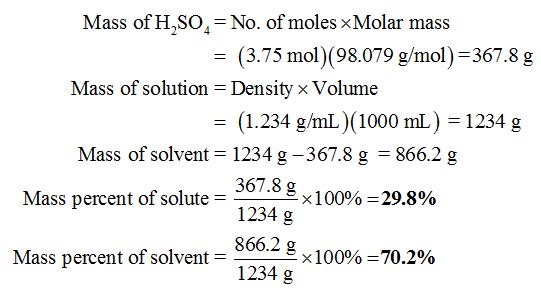Step 2

(b)Calculate molality:

Molality of the solution can be determined using the given formula,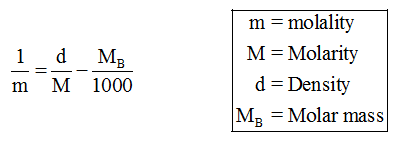Substitute the values as follows,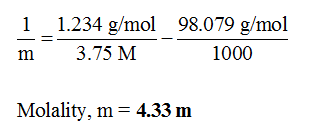Step 3

(c)

Calculate number of moles of solute and solvent: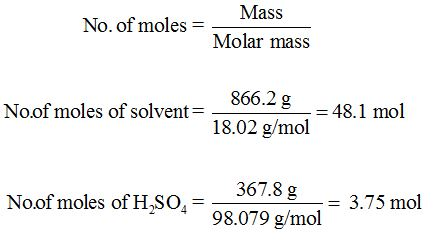...

### Want to see the full answer?

See Solution

#### Want to see this answer and more?

Solutions are written by subject experts who are available 24/7. Questions are typically answered within 1 hour.*

See Solution
*Response times may vary by subject and question.
Tagged in